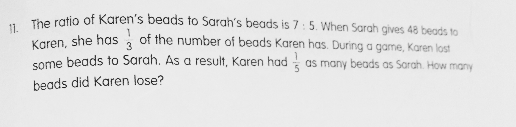# QuestionAnyone help me with attached question

Thanks

7u : 5u : 12u

(5u – 48)/(7u + 48) = 1/3
(5u – 48) x 3 = 15u – 144 ——- 7u + 48
15u – 7u = 8u ——- 48 + 144 = 192
1u ——- 192/8 = 24
7u ——- 7 x 24 = 168 (Karen’s bead before game)
12u ——- 12 x 24 = 288

1 + 5 = 6
288/6 = 48 (Karen’s beads after game)
168 – 48 = 120

0 Replies 1 Like ✔Accepted Answer

First part uses the Constant Total Strategy. Cause even if Sarah passed 48 beads to Karen, their total is the same.

at first,
K : S : Total = > 7 : 5 : 12

After Sarah gave 48 beads to Karen
K : S : Total => 3 : 1 : 4 => 9 : 3 : 12 ,
Highlight in red now share common units, as I made their total the same.

Sarah gave 5u – 3u =2u to Karen,
2u = 48
u =24

Later after the game,
K : S :Total => 1 : 5 : 6 => 2 : 10 : 12 => Constant total strategy again. The total is always the same.

At the game, Karen lost 9u – 2u = 7u = 7 X 24 = 168 beads. #####

0 Replies 1 Like